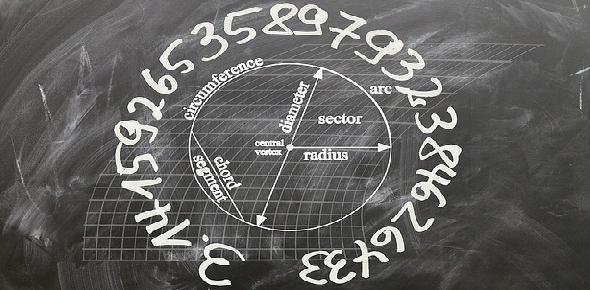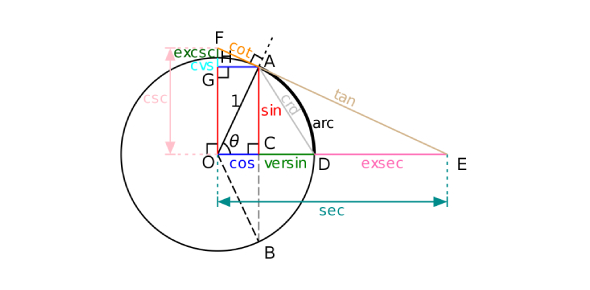What is Euclidean geometry? - ProProfs DiscussTopicsMoreProductsMore+ Ask Question

# What is Euclidean geometry?Change Image    Delete

Asked by K. Tanaka, Last updated: Sep 17, 2020

###Request 0FollowShareAnswer AnonymouslyAnswer LaterCopy Link1W. Pratt, Marketing Analyst, MBA, LincolnEuclidean geometry comes from Greek mathematician Euclid. It is the study of planes and reliable figures. Greek mathematician Euclid first introduced this concept in Elements, which was a textbook he wrote. This study is mainly taught in secondary schools, like college. Many centuries before, when mathematicians referenced geometry, they were referring to Euclidean geometry.This was until the 19th century. After this century, other types of geometry were found from others like Carl Friedrich Gauss and Nikolai Ivanovich Lobachevsky. Euclid made many axioms, which do not need to be provided, including that right angles are congruent and the axiom about parallel postulate.Mike John, Content Explorer, MCA, Los Angeles, California, USA

Hello Guy,

I do not know much about Euclidean Geometry but I agree withJ.Spencer.J.Spencer, Knowledge enthusiast, Tokyo

Euclidean geometry is the study of shapes, sizes, and positions based on the principles and assumptions stated by Greek Mathematician Euclid of Alexandria. It is also called the geometry of flat surfaces. Euclidean geometry is limited to the study of straight lines and objects usually in a 2d space. In practice, Euclidean geometry cannot be applied to curved spaces and curved lines.

Euclid’s geometrical mathematics works under set postulates (called axioms). Euclid’s axioms were considered the base of mathematics related to geometry for almost 2000 years until Einstein’s theory of relativity offset his theories and proved his postulates wrong in relative dimensions of geometry.

The entire bulk of Euclidean geometry is based on 5 axioms or assumptions:

1. A straight line joins two points

2. A straight line can be elongated on both sides to infinity

3. A circle can be made if a point is given as the center and a measure is given for its radius

4. All right angles are equal

5. If a straight line intersects two lines the interior angles on the same sides will always have a sum less than 90∘.

Any system that violates the said axioms comprises non-Euclidean geometry.John F. connor, Content Marketing executive, MA, Minsk,Poland

Euclidean geometry is about the axioms and the theorems associated with these axioms. It was created by the Greek mathematician known and named for Euclid. He was considered to be the father of geometry due to his theorems and thoughts about math. There are methods of proof to his theorems and thoughts. Some of these notions in the book Elements include five main parts. First, things that are equal to each other and equal in whole to the other whole. Second, this one is about Addition property of equality. Third is the subtraction property of equality. The fourth is the reflexive property where one thing equals another thing. Fifth, the entire thing is bigger or more than a part of it.Search for Google imagesSelect a recommended image
Upload from your computerCancelSearch for Google imagesSelect a recommended image
Upload from your computerCancelSearch for Google imagesSelect a recommended image
Upload from your computerCancel